### D.74 Elec­tron spin in a mag­netic field

If you are cu­ri­ous how the mag­netic di­pole strength of the elec­tron can just pop out of the rel­a­tivis­tic Dirac equa­tion, this note gives a quick de­riva­tion.

First, a prob­lem must be ad­dressed. Dirac's equa­tion, chap­ter 12.12, as­sumes that Ein­stein's en­ergy square root falls apart in a lin­ear com­bi­na­tion of terms: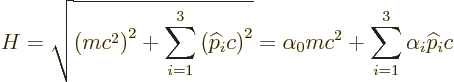which works for the 4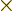4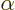ma­tri­ces given in that sec­tion. For an elec­tron in a mag­netic field, ac­cord­ing to chap­ter 13.1 you want to re­place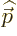with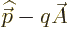where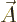is the mag­netic vec­tor po­ten­tial. But where should you do that, in the square root or in the lin­ear com­bi­na­tion? It turns out that the an­swer you get for the elec­tron en­ergy is not the same.

If you be­lieve that the Dirac lin­ear com­bi­na­tion is the way physics re­ally works, and its de­scrip­tion of spin leaves lit­tle doubt about that, then the an­swer is clear: you need to putin the lin­ear com­bi­na­tion, not in the square root.

So, what are now the en­ergy lev­els? That would be hard to say di­rectly from the lin­ear form, so square it down to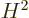, us­ing the prop­er­ties of thema­tri­ces, as given in chap­ter 12.12 and its note. You get, in in­dex no­ta­tion,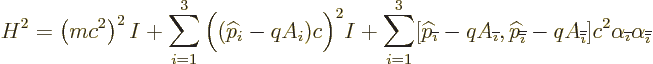where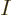is the four by four unit ma­trix,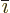is the in­dex fol­low­ing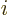in the se­quence 123123..., and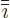is the one pre­ced­ing. The fi­nal sum rep­re­sents the ad­di­tional squared en­ergy that you get by sub­sti­tut­ingin the lin­ear com­bi­na­tion in­stead of the square root. The com­mu­ta­tor arises be­cause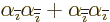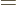0, giv­ing the terms with the in­dices re­versed the op­po­site sign. Work­ing out the com­mu­ta­tor us­ing the for­mu­lae of chap­ter 4.5.4, and the de­f­i­n­i­tion of the vec­tor po­ten­tial,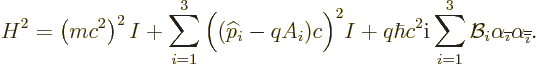By mul­ti­ply­ing out the ex­pres­sions for the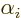of chap­ter 12.12, us­ing the fun­da­men­tal com­mu­ta­tion re­la­tion for the Pauli spin ma­tri­ces that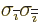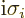,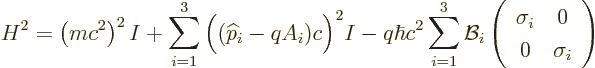It it seen that due to the in­ter­ac­tion of the spin with the mag­netic field, the square en­ergy changes by an amount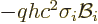. Since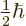times the Pauli spin ma­tri­ces gives the spin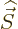, the square en­ergy due to the mag­netic field act­ing on the spin is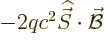.

In the non­rel­a­tivis­tic case, the rest mass en­ergy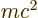is much larger than the other terms, and in that case, if the change in square en­ergy is, the change in en­ergy it­self is smaller by a fac­tor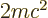, so the en­ergy due to the mag­netic field is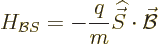(D.52)

which is what was to be proved.